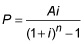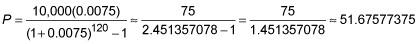##### Finite Math For DummiesA big part of finite math involves working through financial problems. Some of these problems may seem complex—like calculating the monthly deposits required to maintain a sinking fund. Fortunately, there’s a special formula you can use to find the answer.

A sinking fund is usually used to accumulate money to fund a future expense or a way to retire a debt. You can use a sinking fund to pay off a loan in one lump sum at the end of a set amount of time while making just interest payments in the meantime.

For example, a friend borrows \$10,000 to purchase a boat and agrees to pay the full amount back in one payment, ten years from now. In the meantime, he agrees to pay interest monthly on the \$10,000 at an annual rate of 12%. He also sets up a sinking fund to accumulate the lump-sum payment. The sinking fund earns 9% interest, compounded monthly. How much does he pay monthly? The monthly amount is both the interest to the lender and a deposit into the sinking fund.

The interest to the lender is based on an annual rate of 12%. Using the simple interest formula, I = Prt, you have I = 10,000(0.12)(1) = 1,200 per year. Because he plans to make monthly payments, you divide by 12 so \$100 per month goes for the interest payments.

Next, you compute the amount to be deposited in the sinking fund each month.

The formula for a sinking fund payment iswhere P is the amount of the payment, A is the amount to be accumulated, i is the interest rate per time period, and n is the number of time periods.

Using the formula to determine the monthly payment into the sinking fund, the amount, A, is \$10,000, and the interest per pay period is 9% divided by 12, because it’s compounded monthly. The number of time periods over the ten years is 120So, the monthly payment into the sinking fund is about \$51.68. Add that to the interest payments, and the monthly commitment is \$151.68. In ten years, the monthly payments will end.